﻿ An Alternative Proof of a Closed Formula for Central Factorial Numbers of the Second KindPublications are Open
Access in this journal
Article Versions
Export Article
• Normal Style
• MLA Style
• APA Style
• Chicago Style
Research Article
Open Access Peer-reviewed

### An Alternative Proof of a Closed Formula for Central Factorial Numbers of the Second Kind

Feng Qi, Guo-Sheng Wu, Bai-Ni Guo
Turkish Journal of Analysis and Number Theory. 2019, 7(2), 56-58. DOI: 10.12691/tjant-7-2-5
Received March 13, 2019; Revised April 19, 2019; Accepted April 24, 2019

### Abstract

In the short note, by virtue of several formulas and identities for special values of the Bell polynomials of the second kind, the authors provide an alternative proof of a closed formula for central factorial numbers of the second kind. Moreover, the authors pose two open problems on closed form of a special Bell polynomials of the second kind and on closed form of a finite sum involving falling factorials.

2010 Mathematics Subject Classification. Primary 11B83; Secondary 11B75, 33B10.

### 1. Introduction

In mathematics, a closed formula is a mathematical expression that can be evaluated in a finite number of operations. It may contain constants, variables, four arithmetic operations, and elementary functions, but usually no limit.

The Bell polynomials of the second kind, denoted by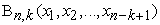for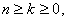are defined 1, 2, 3, 4, 5, 6, 7 by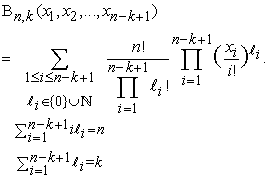The central factorial numbers of the second kind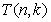for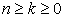can be generated 8, 9, 10 by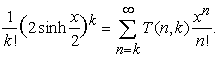(1.1)

The central factorial numbers of the first kind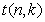forcan be generated 8, 9 by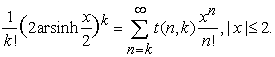(1.2)

In [ 8, Proposition 2.4, (xii)], the authors established the closed formula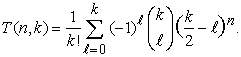(1.3)

In this short note, by virtue of several formulas and identities for special values of the Bell polynomials of the second kind, we will provide an alternative proof of the closed formula (1.3).

### 2. Lemmas

For alternatively proving the closed formula (1.3), we need the following lemmas.

Lemma 2.1 ( 1, 2, 11, 12, 13, 14). The Faà di Bruno formula can be described in terms ofby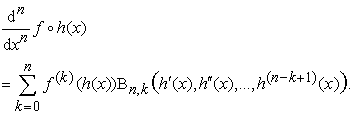(2.1)

For, the Bell polynomials of the second kindsatisfy the identity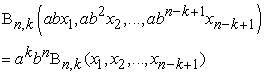(2.2)

is valid, where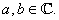Lemma 2.2 10, 11, 12, 14, 15, 16, 17, 18. For, the Bell polynomials of the second kindsatisfy the closed formula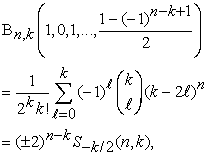(2.3)

where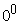is regarded as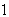and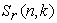denote the associate Stirling numbers of the second kind or weighted Stirling numbers which can be generated by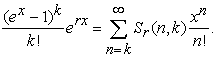### 3. An Alternative Proof of the Closed Formula (1.3)

The closed formula (1.3) can be rewritten in terms of the associate Stirling numbers of the second kind or weighted Stirling numbersas follows.

Theorem 3.1. Forthe central factorial numbers of the second kindsatisfy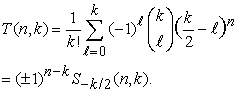(3.1)

Proof. The equation (1.1) implies that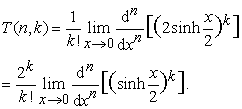(3.2)

Let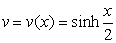. By virtue of the Faà di Bruno formula (2.1), we obtain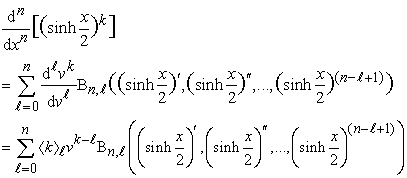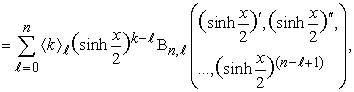where the quantity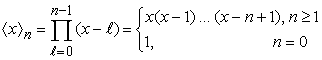is called 2, 19 the falling factorial of x.

Since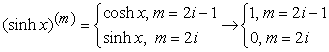for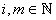as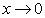, it follows that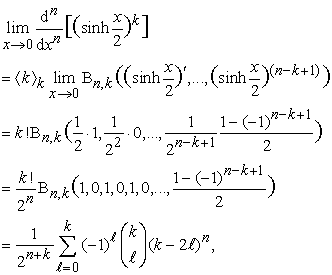where we used the identity (2.2) and the closed formula (2.3). Consequently, the formula (3.1) follows immediately. The proof of Theorem 3.1 is complete.

### 4. Two Open Problems

In this section, we pose two open problems.

4.1. First Open Problem

For alternatively and similarly finding a closed formula for the central factorial numbers of the first kind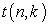generated in (1.2), we need to solve the following open problem.

Open Problem 4.1. Can one find a closed formula of the Bell polynomials of the second kind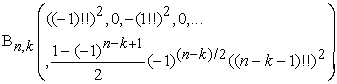for?

4.2. Second Open Problem.

For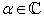and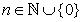, the falling factorial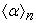is defined by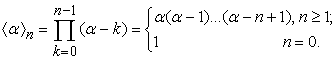(4.1)

In 12, 20 and closely related references therein, the following conclusions were obtained.

Theorem 4.1 ([ 20, Theorem 3.1] and [ 12, Section 1.4]). For, we have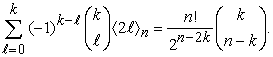(4.2)

Theorem 4.2 ([ 20, Theorem 3.2] and [ 12, Section 1.5]). For, we have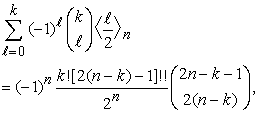(4.3)

where the double factorial of negative odd integers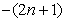for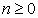is defined by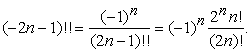The formula (4.3) has been applied in [ 21, Theorem 1.1].

Motivated by the above conclusions, one can naturally pose the following open problem.

Open Problem 4.2. Forand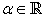, can one find an explicit, elementary, simple, and general formula of the type as in (4.2) and (4.3) for the finite sum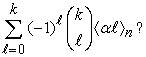In particular, how about special cases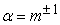for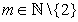in (4.4)?

### References

  C. A. Charalambides, Enumerative Combinatorics, CRC Press Series on Discrete Mathematics and its Applications. Chapman & Hall/CRC, Boca Raton, FL, 2002. In article  L. Comtet, Advanced Combinatorics: The Art of Finite and Infinite Expansions, Revised and Enlarged Edition, D. Reidel Publishing Co., 1974. In article  F. Qi, V. Čerňanová, and Y. S. Semenov, Some tridiagonal determinants related to central Delannoy numbers, the Chebyshev polynomials, and the Fibonacci polynomials, Politehn. Univ. Bucharest Sci. Bull. Ser. A Appl. Math. Phys. 81 (2019), no. 1, 123-136. In article  F. Qi, D. Lim, and B.-N. Guo, Explicit formulas and identities for the Bell polynomials and a sequence of polynomials applied to differential equations, Rev. R. Acad. Cienc. Exactas Fís. Nat. Ser. A Mat. RACSAM 113 (2019), no. 1, 1-9. In article View Article  F. Qi, D. Lim, and Y.-H. Yao, Notes on two kinds of special values for the Bell polynomials of the second kind, Miskolc Math. Notes 20 (2019), no. 1, 465-474. In article View Article  F. Qi and A.-Q. Liu, Alternative proofs of some formulas for two tridiagonal determinants, Acta Univ. Sapientiae Math. 10 (2018), no. 2, 287-297. In article View Article  F. Qi, D.-W. Niu, and B.-N. Guo, Some identities for a sequence of unnamed polynomials connected with the Bell polynomials, Rev. R. Acad. Cienc. Exactas Fís. Nat. Ser. A Math. RACSAM 113 (2019), no. 2, 557-567. In article View Article  P. L. Butzer, M. Schmidt, E. L. Stark, and L. Vogt, Central factorial numbers; their main properties and some applications, Numer. Funct. Anal. Optim. 10 (1989), no. 5-6, 419-488. In article View Article  M. Merca, Connections between central factorial numbers and Bernoulli polynomials, Period. Math. Hungar. 73 (2016), no. 2, 259-264. In article View Article  F. Qi and B.-N. Guo, Relations among Bell polynomials, central factorial numbers, and central Bell polynomials, Math. Sci. Appl. E-Notes 7 (2019), no. 2, 191-194. In article  F. Qi and B.-N. Guo, Explicit formulas for special values of the Bell polynomials of the second kind and for the Euler numbers and polynomials, Mediterr. J. Math. 14 (2017), no. 3, Article 140, 14 pages. In article View Article  F. Qi, D.-W. Niu, D. Lim, and Y.-H. Yao, Special values of the Bell polynomials of the second kind for some sequences and functions, HAL archives (2018), available online at https://hal.archives-ouvertes.fr/hal-01766566. In article  F. Qi and M.-M. Zheng, Explicit expressions for a family of the Bell polynomials and applications, Appl. Math. Comput. 258 (2015), 597-607. In article View Article  C.-F. Wei and F. Qi, Several closed expressions for the Euler numbers, J. Inequal. Appl. 2015, 2015: 219, 8 pages. In article View Article  A. Z. Broder, The r-Stirling numbers, Discrete Math. 49 (1984), no. 3, 241-259. In article View Article  L. Carlitz, Weighted Stirling numbers of the first and second kind, I, Fibonacci Quart. 18 (1980), no. 2, 147-162. In article  M. Griffiths and I. Mezö, A generalization of Stirling numbers of the second kind via a special multiset, J. Integer Seq. 13 (2010), no. 2, Article 10.2.5, 23 pp. In article  B.-N. Guo, I. Mezö, and F. Qi, An explicit formula for the Bernoulli polynomials in terms of the r-Stirling numbers of the second kind, Rocky Mountain J. Math. 46 (2016), no. 6, 1919-1923. In article View Article  F. Qi, X.-T. Shi, and F.-F. Liu, Several identities involving the falling and rising factorials and the Cauchy, Lah, and Stirling numbers, Acta Univ. Sapientiae Math. 8 (2016), no. 2, 282-297. In article View Article  F. Qi, D.-W. Niu, D. Lim, and B.-N. Guo, Closed formulas and identities for the Bell polynomials and falling factorials, Contrib. Discrete Math. 14 (2019), no. 2, in press. In article  F. Qi, Simplifying coefficients in differential equations related to generating functions of reverse Bessel and partially degenerate Bell polynomials, Bol. Soc. Paran. Mat. 39 (2021), no. 4, in press. In article

Published with license by Science and Education Publishing, Copyright © 2019 Feng Qi, Guo-Sheng Wu and Bai-Ni GuoThis work is licensed under a Creative Commons Attribution 4.0 International License. To view a copy of this license, visit http://creativecommons.org/licenses/by/4.0/